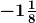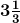# Find the multiplicative inverse of

Question:

Find the multiplicative inverse of

(i) $-1 \frac{1}{8}$

(ii) $3 \frac{1}{3}$

Solution:

(i)The given numbercan be written as = -9/8

The multiplicative inverse = -8/9

(ii)The given numbercan be written as = 10/3

The multiplicative inverse = 3/10